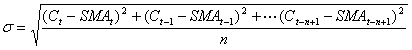Chart Pro online
User name (email):

 Recently used

 Forex

 Indices

# Standard Deviation (SMD)

Standard deviation (SMD) is statistical measure of volatility.

Standard deviation is calculated by formula:Standard deviation is calculated for closing prices. For average value is used simple moving average (SMA). Default value for parameter n is 21.Standard Deviation (SMD)

Interpretation

Standard deviation is not indicator which is used for creating buying and selling signals. It is used for calculation of another indicator in technical analysis: bollinger bands.

Example